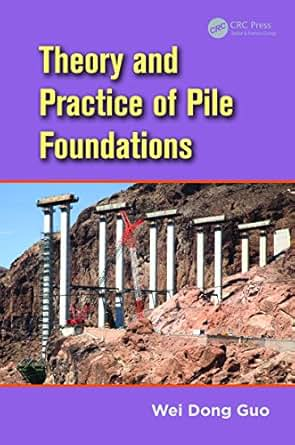## Sunday, October 21, 2018

### Theory And Practice Of Pile Foundation (Free PDF File)Content :-

1 Strength and stiffness from in situ tests :-
1.1 Standard penetration tests (SPT).
1.2 Cone penetration tests.
1.3 Soil stiffness.
1.4 Stiffness and strength of rock.

2 Capacity of vertically loaded piles :-
2.1 Introduction.
2.2 Capacity of single piles.
2.3 Capacity of single piles in rock.
2.4 Negative skin friction.
2.5 Capacity of pile groups.

3 Mechanism and models for pile soil interaction :-
3.1 Concentric cylinder model (CCM).
3.2 Nonlinear concentric cylinder model.
3.3 Time-dependent CCM.
3.4 Torque-rotation transfer model.
3.5 Coupled elastic model for lateral piles.
3.6 Elastic-plastic model for lateral piles.

4 Vertically loaded single piles :-
4.1 Introduction.
4.3 Overall pile–soil interaction.
4.4 Paramatric study.
4.6 Settlement influence factor.
4.7 Summary.
4.8 Capacity for strain-softening soil.
4.9 Capacity and cyclic amplitude.

5 Time-dependent response of vertically loaded piles :-
5.2 Visco-elastic consolidation.

6 Settlement of pile groups :-
6.1 Introduction
6.2 Empirical methods.
6.3 Shallow footing analogy.
6.4 Numerical methods.
6.5 Boundary element approach: GASGROUP.

7 Elastic solutions for laterally loaded piles :-
7.1 Introduction
7.2 Overall pile response
7.3 Validation
7.4 Parametric study
7.5 Subgrade modulus and design charts
7.6 Pile group response
7.7 Conclusion.

8 Laterally loaded rigid piles :-
8.1 Introduction.
8.2 Elastic plastic solutions.
8.4 Comparison with existing solutions.
8.5 Illustrative examples.
8.6 Summary.

9.1 Introduction.
9.2 Solutions for pile–soil system.
9.3 Slip depth versus nonlinear response.
9.4 Calculations for typical piles.
9.5 Comments on use of current solutions.
9.8 Summary.

10 Structural nonlinearity and response of rock-socket piles :-
10.1 Introduction.
10.2 Solutions for laterally loaded shafts.
10.3 Nonlinear structural behavior of shafts.
10.4 Nonlinear piles in sand/clay.
10.5 Rock-socketed shafts.
10.6 Conclusion.

11 Laterally loaded pile groups :-
11.1 Introduction.
11.2 Overall solutions for a single pile.
11.3 Nonlinear response of single piles and pile groups.
11.4 Examples.
11.5 Conclusions.

12 Design of passive piles :-
12.1 Introduction.
12.2 Mechanism for passive pile–soil interaction.
12.3 Elastic-plastic (EP) solutions.
12.4 pu-based solutions (rigid piles).
12.5 E-E, EP-EP solutions (deep sliding–flexible piles).
12.6 Design charts.
12.7 Case study..
12.8 Conclusion.

13 Physical modeling on passive piles :-
13.1 Introduction.
13.2 Apparatus and test procedures.
13.3 Test results.
13.4 Simple solutions.
13.5 Conclusion.